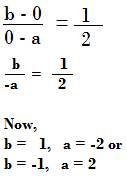## Applications of slope

Slope can be used in the following ways

Suppose three points are given.

If the slope of any two pairs of points is same, then the points lie on a same straight line. Therefore, the points are collinear.

Let the three points be

A (2, 4)   B (4, 6) C (6, 8)

Now slope of the two points AB is

Slope of the two points BC is

Slope of the two points AC is

Slope of a straight line is a unique value. It does not change whichever two points lying on it are taken.

Since, the slope of any two pairs of points are same, the above three points lie on a same straight line.

Therefore, the three given points A (2, 4)   B (4, 6) C (6, 8) are collinear.

Slopes are used to check if two straight lines are parallel or perpendicular.

To use slope for this, equations of two straight lines will be given.

Consider the following equations of two straight lines

3x + 4y + 5 = 0

6x + 8y – 9 = 0

Slope of the first straight line is -3/4

Slope of the second straight line is -6/8 = -3/4

Since slopes are equal, therefore the two straight lines whose equations are as given above are parallel to each other.

To compare x intercept and y intercept of a straight line, one of the methods is to use slope of the line.

Consider the straight line L with x intercept ‘a’ and y intercept ‘b’

Let slope of the straight line be ½.

We are now, curious to know which of the two intercepts, the x intercept ‘a’ or the y intercept ‘b’ is greater?

Slope of the line L passing through the two points

A (a, 0) and B (0, b) is

Since slope is ½, thereforeTherefore, either of the intercepts is greater based on slope. An additional information is required to exactly say which of the two intercepts is always greater.